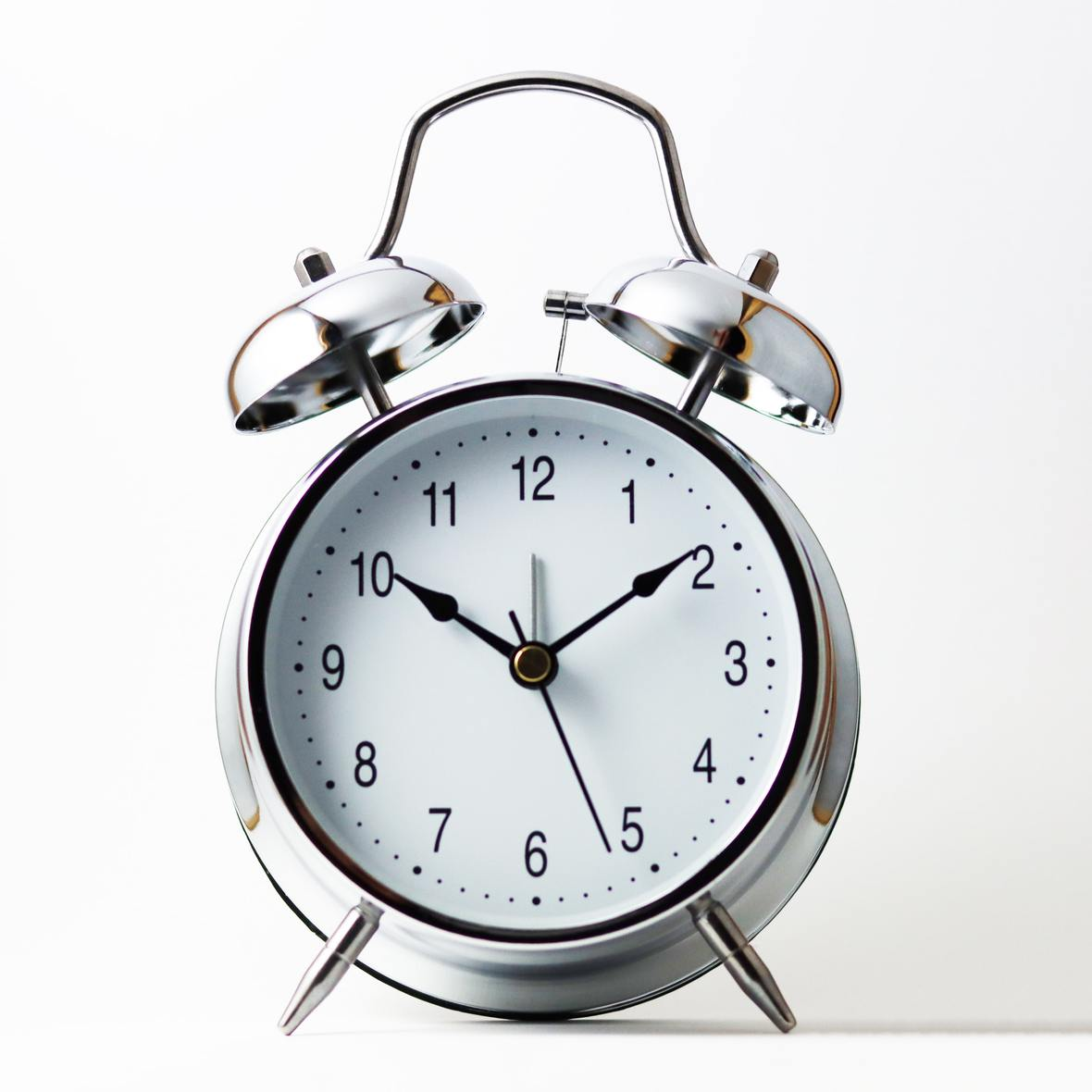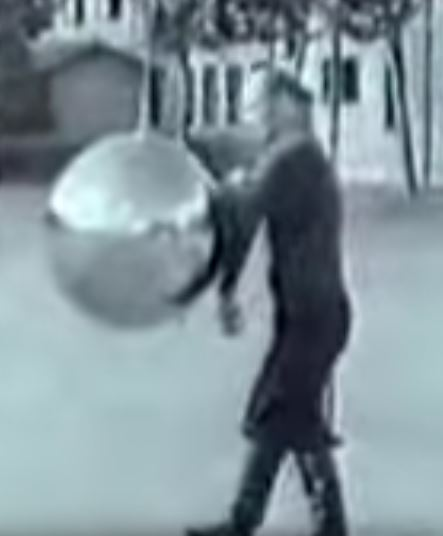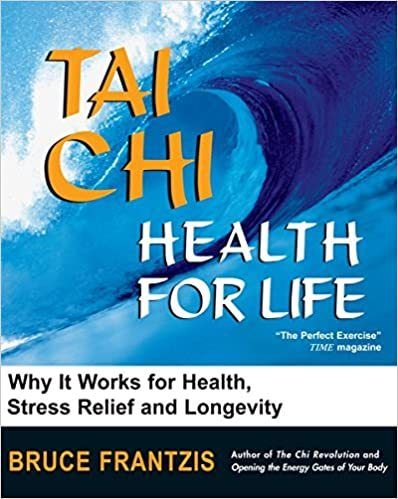Like   Tweet   Pin   +1   in/* styles */ Performance, Wellbeing, Tai Chi, Tips and Resources   ||   Issue #31 October 2020
 table div table+table+table div table{width:100%;padding:0}table div table+table+table div table img{width:96.23%;padding:0;float:none}table div table+table+table div table td{width:100%;padding:0 1.88% 18px}/* styles */Welcome to Good Chi News and in this issue you'll find Tai Chi Tips, a short interview of me discussing students' questions, a clip on early Wu Style training, a recommended Tai Chi book and an ancient quote by Lao Tzu.

Regards
Chris Bennett
Chris Chi

 table div table+table+table+table+table div table{width:100%;padding:0}table div table+table+table+table+table div table img{width:96.23%;padding:0;float:none}table div table+table+table+table+table div table td{width:100%;padding:0 1.88% 18px}/* styles */## Wake Up CallA good way of waking the mind and body up is to do at least one Tai Chi or Chi Kung posture eight times.

This takes very little time and effort and it's a great energiser that can be used anytime during the day.

So when your internal alarm clock sounds to indicate you're tired and/or unfocused, turn it off by turning yourself onto a bit of Tai Chi or Chi Kung.

Image courtesy of Joan Tan via Unsplash.

 table div table+table+table+table+table+table+table+table div table{width:100%;padding:0}table div table+table+table+table+table+table+table+table div table img{width:96.23%;padding:0;float:none}table div table+table+table+table+table+table+table+table div table td{width:100%;padding:0 1.88% 18px}/* styles */## Tai Chi Tip

 /* styles */ When practicing your Tai Chi form focus on one theme at a time. E.g breathing or weighting etc.
 table div table+table+table+table+table+table+table+table+table+table+table div table{width:100%;padding:0}table div table+table+table+table+table+table+table+table+table+table+table div table img{width:96.23%;padding:0;float:none}table div table+table+table+table+table+table+table+table+table+table+table div table td{width:100%;padding:0 1.88% 18px}/* styles */## My ThoughtsHere's a short informal interview of me conducted by Neil R. Hall, Director of Internal Arts and LCTKD International.

He asked me three questions for his junior students:
1. What age where you when you started Tai Chi?
2. Is there anything you still want to achieve?
3. What is different between martial arts in the UK and Australia?

This interview was held in England last year when I was on holidays.

 table div table+table+table+table+table+table+table+table+table+table+table+table+table+table div table{width:100%;padding:0}table div table+table+table+table+table+table+table+table+table+table+table+table+table+table div table img{width:96.23%;padding:0;float:none}table div table+table+table+table+table+table+table+table+table+table+table+table+table+table div table td{width:100%;padding:0 1.88% 18px}/* styles */## Early Wu Style TrainingIn this 1937 clip Chu Minyi, a disciple of Wu Jianquan (founder of Wu Style) demonstrates the Wu Styke form which he performs quickly, push hands, and various training methods.

In one of the training methods I like the way he continually kicks a shuttlecock up in the air without letting it hit the ground (7.46)

The quality of the clip is poor but it's still worth a look.

 table div table+table+table+table+table+table+table+table+table+table+table+table+table+table+table+table+table div table{width:100%;padding:0}table div table+table+table+table+table+table+table+table+table+table+table+table+table+table+table+table+table div table img{width:96.23%;padding:0;float:none}table div table+table+table+table+table+table+table+table+table+table+table+table+table+table+table+table+table div table td{width:100%;padding:0 1.88% 18px}/* styles */## Tai Chi Health For LifeTai Chi Health For Life authored by Bruce Frantzis is a good read for those interested in learning about Tai Chi.

It's also ideal for those considering learning Tai Chi as it gives a good overall introduction to the art.

You can purchase the book on Amazon

 table div table+table+table+table+table+table+table+table+table+table+table+table+table+table+table+table+table+table+table+table div table{width:100%;padding:0}table div table+table+table+table+table+table+table+table+table+table+table+table+table+table+table+table+table+table+table+table div table img{width:96.23%;padding:0;float:none}table div table+table+table+table+table+table+table+table+table+table+table+table+table+table+table+table+table+table+table+table div table td{width:100%;padding:0 1.88% 18px}/* styles */## What's NewI'm considering revamping my Tai Chi book and also creating an ebook from it. I published it in 1999 and it's time to update it.

A realistic estimate of completing this project and have it available for purchase would be sometime early to mid next year.

My Tai Chi book is an illustrated workbook that covers the first fourteen movements of Wu Style Tai Chi and is ideal for beginners and/or those who are looking for a short form to learn.

If you are interested in purchasing a book or ebook let me know and I'll contact you when it's published.

Image courtesy of Genevieve Peron-Migneron via Unsplash

 table div table+table+table+table+table+table+table+table+table+table+table+table+table+table+table+table+table+table+table+table+table+table+table div table{width:100%;padding:0}table div table+table+table+table+table+table+table+table+table+table+table+table+table+table+table+table+table+table+table+table+table+table+table div table img{width:96.23%;padding:0;float:none}table div table+table+table+table+table+table+table+table+table+table+table+table+table+table+table+table+table+table+table+table+table+table+table div table td{width:100%;padding:0 1.88% 18px}/* styles */table div table+table+table+table+table+table+table+table+table+table+table+table+table+table+table+table+table+table+table+table+table+table+table+table+table div table{width:100%;padding:0}table div table+table+table+table+table+table+table+table+table+table+table+table+table+table+table+table+table+table+table+table+table+table+table+table+table div table img{width:96.23%;padding:0;float:none}table div table+table+table+table+table+table+table+table+table+table+table+table+table+table+table+table+table+table+table+table+table+table+table+table+table div table td{width:100%;padding:0 1.88% 18px}/* styles *//* styles */
 table div table+table+table+table+table+table+table+table+table+table+table+table+table+table+table+table+table+table+table+table+table+table+table+table+table+table+table div table{width:100%;padding:0}table div table+table+table+table+table+table+table+table+table+table+table+table+table+table+table+table+table+table+table+table+table+table+table+table+table+table+table div table img{width:96.23%;padding:0;float:none}table div table+table+table+table+table+table+table+table+table+table+table+table+table+table+table+table+table+table+table+table+table+table+table+table+table+table+table div table td{width:100%;padding:0 1.88% 18px}/* styles *//* styles */